# Resampling Methods

waldronlab.github.io / waldronlab.org

June 15, 2017

# Outline and introduction

• Objectives: prediction or inference?
• Cross-validation
• Bootstrap
• Permutation Test
• Monte Carlo Simulation

ISLR Chapter 5: James, G. et al. An Introduction to Statistical Learning: with Applications in R. (Springer, 2013). This book can be downloaded for free at http://www-bcf.usc.edu/~gareth/ISL/getbook.html

# Why do regression?

Inference
• Questions:
• Which predictors are associated with the response?
• How are predictors associated with the response?
• Example: do dietary habits influence the gut microbiome?
• Linear regression and generalized linear models are the workhorses
• We are more interested in interpretability than accuracy
• Produce interpretable models for inference on coefficients

Bootstrap, permutation tests

# Why do regression? (cont’d)

Prediction
• Questions:
• How can we predict values of $$Y$$ based on values of $$X$$
• Examples: Framingham Risk Score, OncotypeDX Risk Score
• Regression methods are still workhorses, but also less-interpretable machine learning methods
• We are more interested in accuracy than interpretability
• e.g. sensitivity/specificity for binary outcome
• e.g. mean-squared prediction error for continuous outcome

Cross-validation

# Why cross-validation?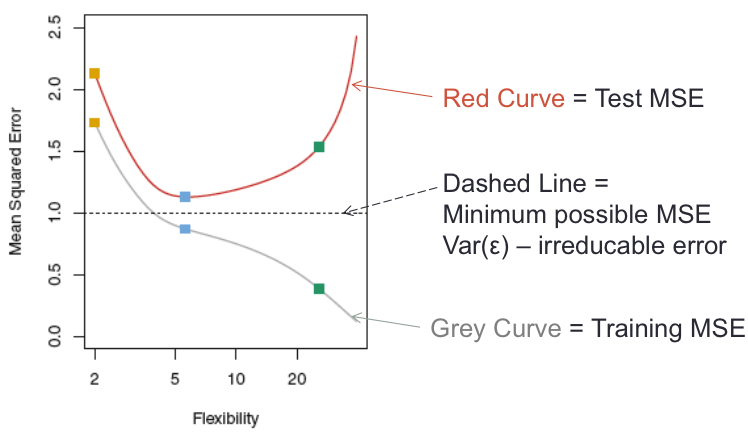Figure 2.9 B

Under-fitting, over-fitting, and optimal fitting

# K-fold cross-validation approach

• Create $$K$$ “folds” from the sample of size $$n$$, $$K \le n$$
1. Randomly sample $$1/K$$ observations (without replacement) as the validation set
2. Use remaining samples as the training set
3. Fit model on the training set, estimate accuracy on the validation set
4. Repeat $$K$$ times, not using the same validation samples
5. Average validation accuracy from each of the validation sets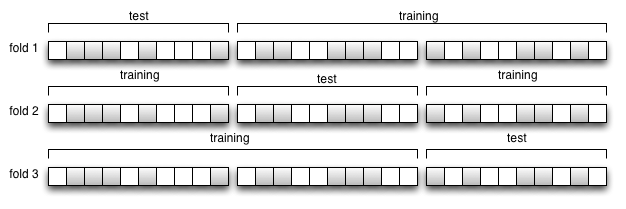3-fold CV

# Variability in cross-validation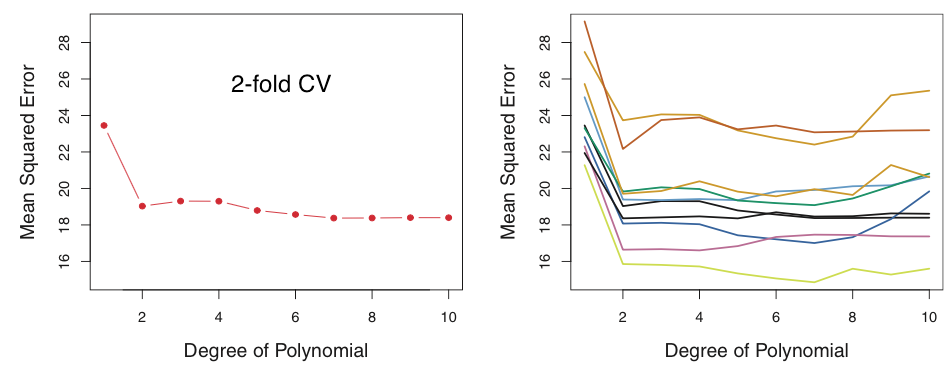Variability of 2-fold cross-validation (ISLR Figure 5.2)

# Cross-validation summary

• In prediction modeling, we think of data as training or test
• Cross-validation estimates test set error from a training set
• Training set error always decreases with more complex (flexible) models
• Test set error as a function of model flexibility tends to be U-shaped
• The low point of the U represents the optimal bias-variance trade-off, or the most appropriate amount of model flexibility
• Computationally, $$K$$ models must be fitted
• 5 or 10-fold CV are popular choices
• can be repeated for smoothing (e.g. see Braga-Neto et al 2004. Is cross-validation valid for small-sample microarray classification?. Bioinformatics, 20(3), 374-380.)

# Cross-validation caveats

• Be very careful of information “leakage” into test sets, e.g.:
• feature selection using all samples
• “human-loop” over-fitting
• changing your mind on accuracy measure
• try a different dataset

http://hunch.net/?p=22

# Cross-validation caveats (cont’d)

• Tuning plus accuracy estimation requires nested cross-validation
• Example: high-dimensional training and test sets simulated from identical true model
• Penalized regression models tuned by 5-fold CV
• Tuning by cross-validation does not prevent over-fitting

Waldron et al.: Optimized application of penalized regression methods to diverse genomic data. Bioinformatics 2011, 27:3399–3406.

# Cross-validation caveats (cont’d)

• Cross-validation estimates assume that the sample is representative of the population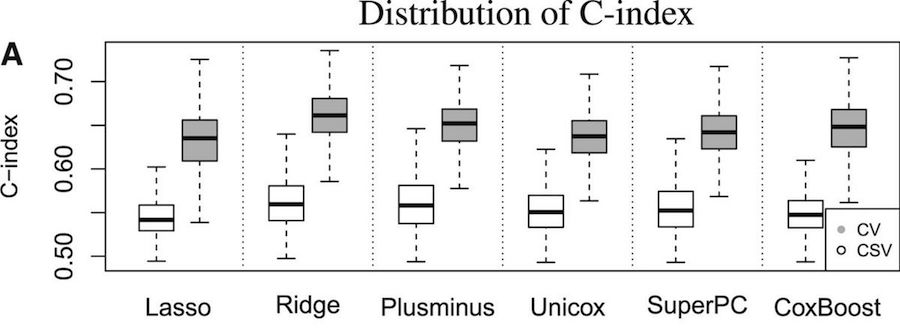Cross-validation vs. cross-study validation in breast cancer prognosis

Bernau C et al.: Cross-study validation for the assessment of prediction algorithms. Bioinformatics 2014, 30:i105–12.

# Permutation test

• Classical hypothesis testing: $$H_0$$ of test statistic derived from assumptions about the underlying data distribution
• e.g. $$t$$, $$\chi^2$$ distribution
• Permutation testing: $$H_0$$ determined empirically using permutations of the data where $$H_0$$ is guaranteed to be true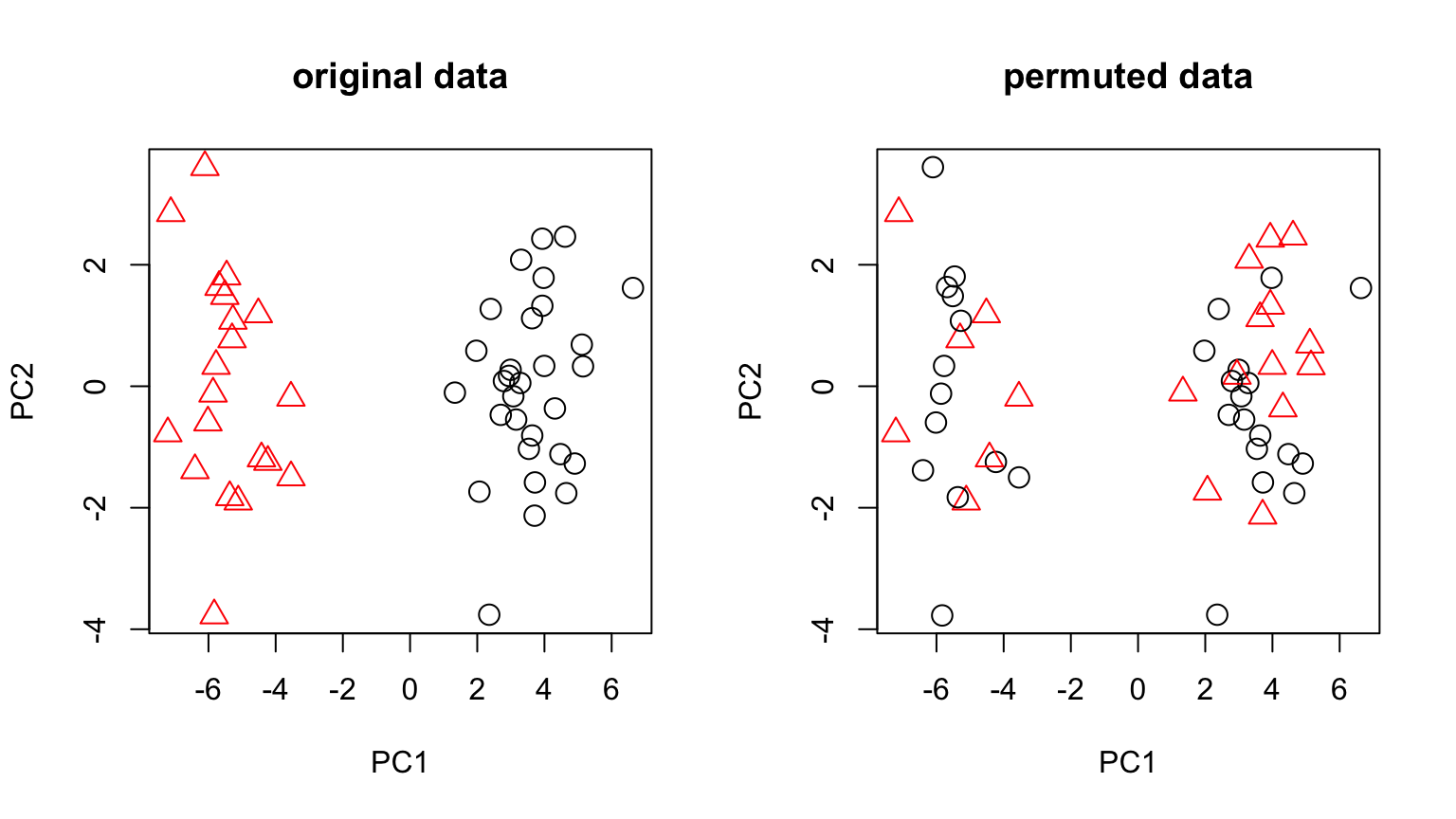# Steps of permutation test:

1. Calculate test statistic (e.g. T) in observed sample
2. Permutation:
1. Sample without replacement the response values ($$Y$$), using the same $$X$$
2. re-compute and store the test statistic T
3. Repeat R times, store as a vector $$T_R$$
3. Calculate empirical p value: proportion of permutation $$T_R$$ that exceed actual T

# Calculating a p-value

$P = \frac{sum \left( abs(T_R) > abs(T) \right)+ 1}{length(T_R) + 1}$

• Phipson B, Smyth GK: Permutation P-values should never be zero: calculating exact P-values when permutations are randomly drawn. Stat. Appl. Genet. Mol. Biol. 2010, 9:Article39.

# Calculating a False Discovery Rate

• calculate # all discoveries from unpermuted data
• estimate # false discoveries by averaging over permutations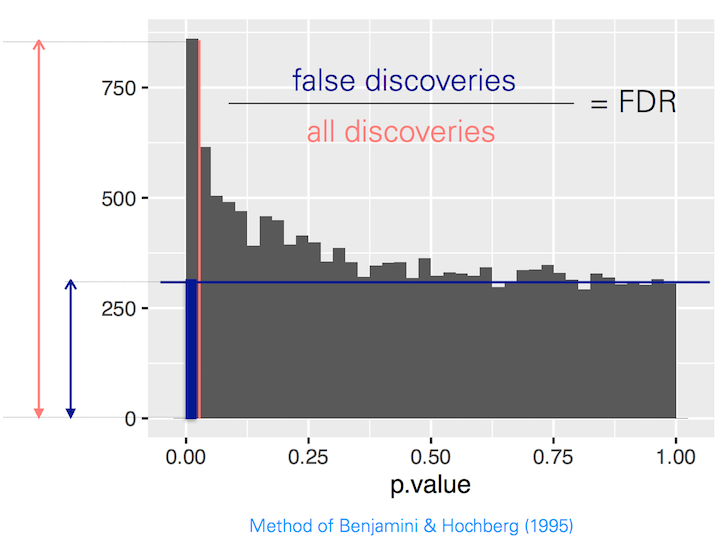# Permutation test - pros and cons

• Pros:
• does not require distributional assumptions
• can be applied to any test statistic
• applicable to False Discovery Rate estimation
• Cons:
• less useful for small sample sizes
• in naive implementations, can get p-values of “0”

# Example from (sleep) data:

• Sleep data show the effect of two soporific drugs (increase in hours of sleep compared to control) on 10 patients.
##      extra        group        ID
##  Min.   :-1.600   1:10   1      :2
##  1st Qu.:-0.025   2:10   2      :2
##  Median : 0.950          3      :2
##  Mean   : 1.540          4      :2
##  3rd Qu.: 3.400          5      :2
##  Max.   : 5.500          6      :2
##                          (Other):8

# t-test for difference in mean sleep

##
##  Welch Two Sample t-test
##
## data:  extra by group
## t = -1.8608, df = 17.776, p-value = 0.07939
## alternative hypothesis: true difference in means is not equal to 0
## 95 percent confidence interval:
##  -3.3654832  0.2054832
## sample estimates:
## mean in group 1 mean in group 2
##            0.75            2.33

# Permutation test instead of t-test

set.seed(1)
permT = function(){
index = sample(1:nrow(sleep), replace=FALSE)
t.test(extra ~ group[index], data=sleep)$statistic } Tr = replicate(999, permT()) (sum(abs(Tr) > abs(Tactual)) + 1) / (length(Tr) + 1) ##  0.079 # Bootstrap # The Bootstrap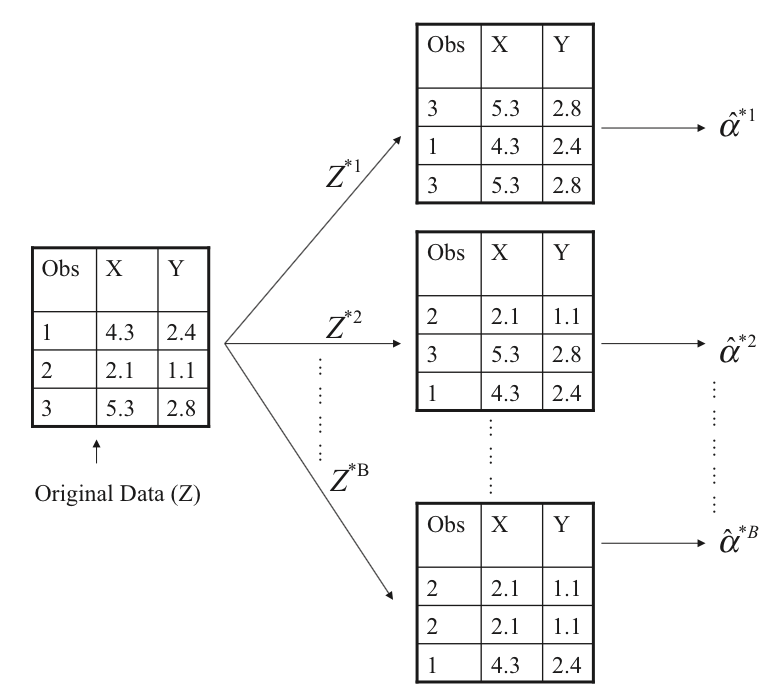Schematic of the Bootstrap ISLR Figure 5.11: Schematic of the bootstrap # Uses of the Bootstrap • The Bootstrap is a very general approach to estimating sampling uncertainty, e.g. standard errors • Can be applied to a very wide range of models and statistics • Robust to outliers and violations of model assumptions # How to perform the Bootstrap • The basic approach: 1. Using the available sample (size $$n$$), generate a new sample of size $$n$$ (with replacement) 2. Calculate the statistic of interest 3. Repeat 4. Use repeated experiments to estimate the variability of your statistic of interest # Example: bootstrap in the sleep dataset • We used a permutation test to estimate a p-value • We will use bootstrap to estimate a confidence interval t.test(extra ~ group, data=sleep) ## ## Welch Two Sample t-test ## ## data: extra by group ## t = -1.8608, df = 17.776, p-value = 0.07939 ## alternative hypothesis: true difference in means is not equal to 0 ## 95 percent confidence interval: ## -3.3654832 0.2054832 ## sample estimates: ## mean in group 1 mean in group 2 ## 0.75 2.33 # Example: bootstrap in the sleep dataset set.seed(2) bootDiff = function(){ boot = sleep[sample(1:nrow(sleep), replace = TRUE), ] mean(boot$extra[boot$group==1]) - mean(boot$extra[boot\$group==2])
}
bootR = replicate(1000, bootDiff())
bootR[match(c(25, 975), rank(bootR))]
##  -3.32083333  0.02727273

note: better to use library(boot)

# Example: oral carcinoma recurrence risk

• Oral carcinoma patients treated with surgery
• Surgeon takes “margins” of normal-looking tissue around to tumor to be safe
• number of “margins” varies for each patient
• Can an oncogenic gene signature in histologically normal margins predict recurrence?

Reis PP, Waldron L, et al.: A gene signature in histologically normal surgical margins is predictive of oral carcinoma recurrence. BMC Cancer 2011, 11:437.

# Example: oral carcinoma recurrence risk

• Model was trained and validated using the maximum expression of each of 4 genes from any margin